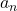## Find the 5th term of the arithmetic sequence with a7=25 and a3 =55.

Question

Find the 5th term of the arithmetic sequence with a7=25 and a3 =55.

in progress 0
6 months 2021-07-28T02:55:33+00:00 1 Answers 6 views 0

a₅ = 40

Step-by-step explanation:

The n th term of an arithmetic sequence is= a₁ + (n – 1)d

where a₁ is the first term and d the common difference

Given a₇ = 25 and a₃ = 55 , then

a₁ + 2d = 55 → (1)

a₁ + 6d = 25 → (2)

Subtract (1) from (2)  term by term to eliminate a₁, then

4d = – 30 ( divide both sides by 4 )

d = – 7.5

Substitute d = 7.5 into (1)

a₁ + 2(- 7.5) = 55

a₁ – 15 = 55 ( add 15 to both sides )

a₁ = 70

Thus

a₅ = 70 – 7.5(4) = 70 – 30 = 40